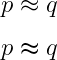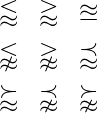# How to write a approximately equal symbol(≈) in LaTeX?

Mathematically an approximately equal symbol is formed by two similar to symbols. And the approximate symbol is denoted by the \approx and \thickapprox commands.

\documentclass{article}
\usepackage{amssymb}
\begin{document}
$p \approx q$
$p \thickapprox q$
\end{document}

Output :Notice in the program above that the amssymb package has been used for the \thickapprox command.

You can also use more than one symbol with approximate symbols. For example equals, less than, grater than, etc.

\documentclass{article}
\usepackage{amssymb}
\begin{document}
$\begin{matrix} \lessapprox & \gtrapprox & \approxeq \\[6pt] \lnapprox & \gnapprox & \precapprox \\[6pt] \succapprox & \precnapprox & \succnapprox \end{matrix}$
\end{document}

Output :However, to represent each of the above symbols you need to take the help of the amssymb package.#### Md Jidan Mondal

LaTeX expert with over 10 years of experience in document preparation and typesetting. Specializes in creating professional documents, reports, and presentations using LaTeX.